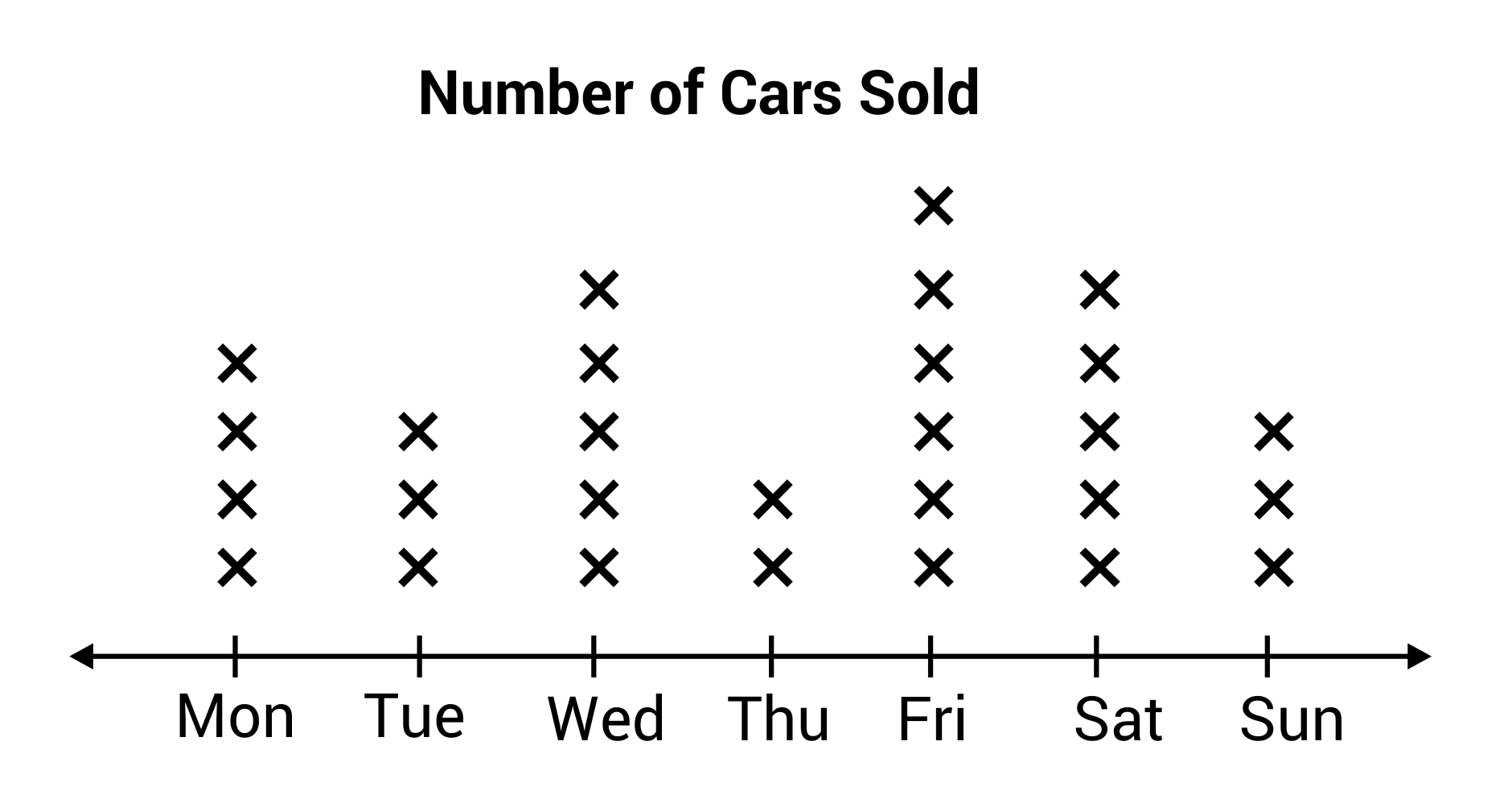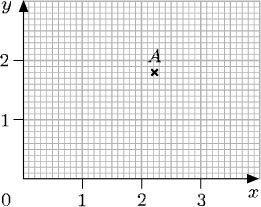Mixed Numbers
Polygons
Number Stories
Data
Division
100

This the correct answer to 1/2 x 5

2 and 1/2

100

This is the most specific type of quadrilateral.

square

100

Timmy has 4 pounds of clay that his is breaking into 1/3 pound pieces. This is how many pieces he will have.

12 pieces

100This is the day that the most cars were sold.

Friday

100

This is the correct answer to 4 divided by 1/2.

8

200

This is the correct answer to 5 x 1/2.

2 and 1/2

200

A parallelogram can also be called these 2 names.

200

This is the area of a rug that is 2 1/4 feet by 5 feet.

11 and 1/4 feet squared

200How many cars were sold on the weekend? (Sat & Sun)

8 cars

200

This is the correct answer to 1/2 divided by 4

1/8

300

This is the correct answer to 2 and 1/2 x 2 and 1/2.

6 and 1/4

300

These are the properties of a kite.

All of the properties of a quadrilateral with 2 pairs of adjacent equal-length sides.

300

This is the area of a yard that is 5 meters long and 8.5 meters wide.

42.5 square meters

300If this line plot shows plant height, what is the total number of plants that grew less than 1 inch?

10 plants

300

This is the correct answer to 1/4 divided 7

1/28

400

This is the correct answer to 2 x 4 and 5/8.

9 and 2/8

400

These are the properties that make squares and rectangles unique.

Right angles

400

Write a number story with the equation 2 divided by 1/3.

400If this line plot show plant height, what is the total height of all of the plants that grew less than an inch?

4 and 5/8 inches

400

Use common denominators to solve 7 divided by 1/3.

21/3 divided by 1/3 = 21

500

This is the correct answer to 5 and 1/3 x 2 and 1/4.

12

500

This is the definition of HIERARCHY.

A system that organizes or ranks things according to their characteristics.

500

Write and solve a number story for 2 and 1/4 x 5 and 4/5.

500What is the location of point A?

(2 and 2/10, 1 and 8/10)

500

Use common denominators to solve 1/5 divided by 3.

1/5 divided by 15/5 = 1/15

Click to zoom